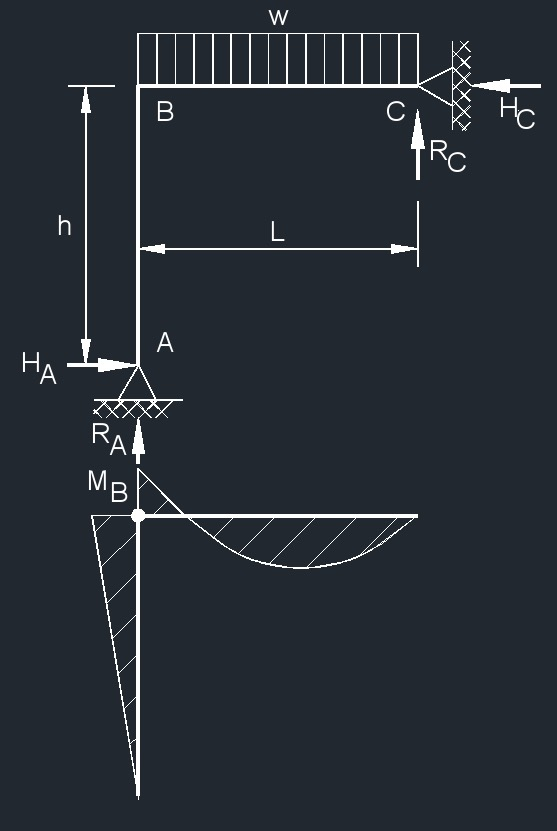# Two Member Frame - Pin/Pin Top Uniformly Distributed Load

Written by Jerry Ratzlaff on . Posted in Structural

###Two Member Frame - Pin/Pin Top Uniformly Distributed Load Formula

$$\large{ e = \frac{h}{L} }$$

$$\large{ \beta = \frac{I_h}{I_v} }$$

$$\large{ R_A = \frac{w\;L}{8} \; \left( \frac{ 4\; \beta \; e \;+\; 5 }{\beta \; e \;+\; 1} \right) }$$

$$\large{ R_C = \frac{w\;L}{8} \; \left( \frac{ 4\; \beta \; e \;+\; 3 }{\beta \; e \;+\; 1} \right) }$$

$$\large{ H_A = H_C = \frac{ w\;L^2 }{ 8\;h\; \left( \beta \; e \;+\; 1 \right) } }$$

$$\large{ H_B = \frac{ w\;L^2 }{ 8\; \left( \beta \; e \;+\; 1 \right) } }$$

Where:

$$\large{ h }$$ = height of frame

$$\large{ x }$$ =  horizontal distance from reaction point

$$\large{ H }$$ =  horizontal reaction load at bearing point

$$\large{ w }$$ = load per unit length

$$\large{ M }$$ = maximum bending moment

$$\large{ A, B, C }$$ = points of intersection on frame

$$\large{ R }$$ = reaction load at bearing point

$$\large{ I }$$ = second moment of area (moment of inertia)

$$\large{ I_h }$$ = horizontal second moment of area (moment of inertia)

$$\large{ I_v }$$ = vertical second moment of area (moment of inertia)

$$\large{ L }$$ = span length of the bending member